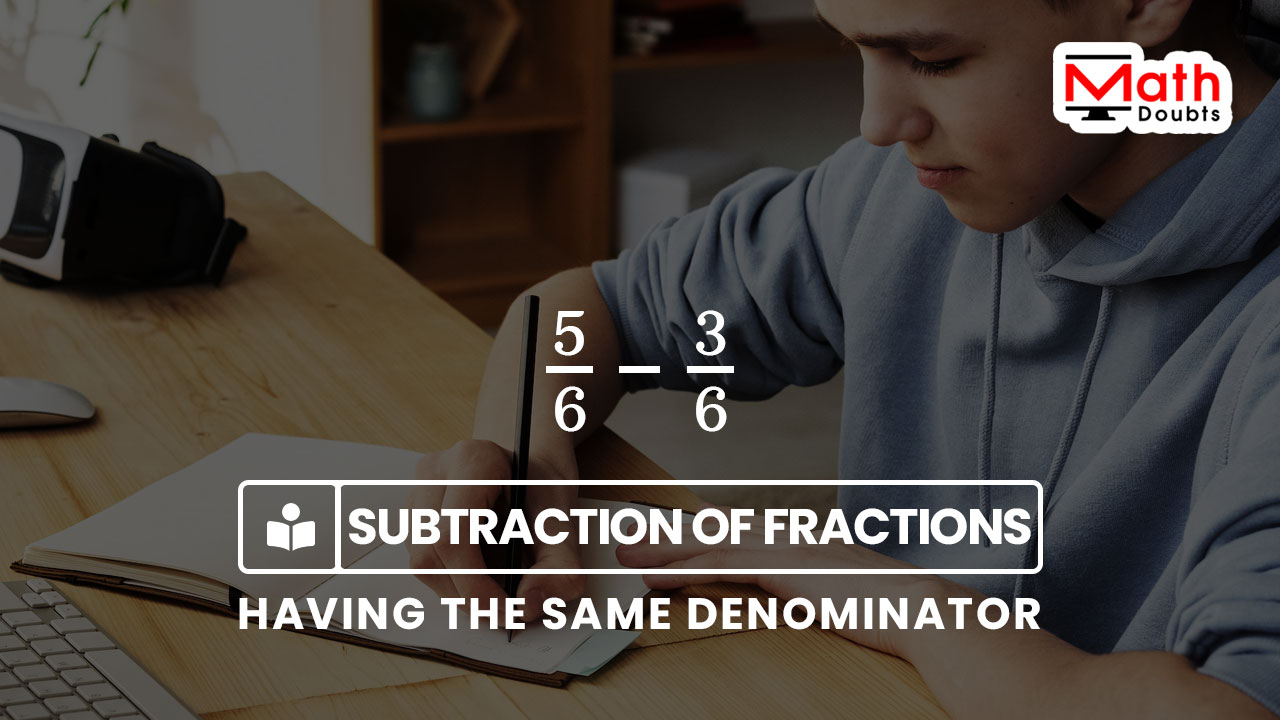# Subtraction of the fractions with the same denominator

## Definition

The mathematical operation of subtracting a fraction from another fraction having same denominator is called the subtraction of the like fractions.

### Introduction

A minus sign is placed between two fractions whose denominator is same. It expresses that one fraction should be subtracted from its like fraction to find the difference between them in mathematics. The mathematical process of finding the difference between the fractions that contain the same denominator is called the subtraction of the like fractions.There are three simple steps to find the difference of the fractions, which consist of the same denominator.

1. Write the two fractions having the same denominator in a row by placing a minus sign between them.
2. The denominators of both fractions are the same in this case. So, the denominator for their difference should also be same but the numerator for their difference is the difference of their numerators.
3. Finally, subtract the numbers in the numerator to find the difference of the like fractions.

Now, let’s learn how to subtract a fraction from its like fraction by following the above three steps.

#### Example

Subtract the fractions $\dfrac{1}{5}$ and $\dfrac{3}{5}$

Firstly, write the two fractions in a row but include a minus sign between them to express the subtraction of the fractions with the same denominator in mathematical form.

$=\,\,$ $\dfrac{3}{5}$ $-$ $\dfrac{1}{5}$

The expression expresses that the denominator of every fraction is same and it is $5$ in this example. So, the denominator for the difference between them should also be equal to $5$. Due to the equal denominators, the numerator of their difference is the difference of their numerators.

$\implies$ $\dfrac{3}{5}$ $-$ $\dfrac{1}{5}$ $\,=\,$ $\dfrac{3-1}{5}$

Now, subtract the number $1$ from the number $3$ to find the difference between them in the numerator.

$\,\,\,\therefore\,\,\,\,\,\,$ $\dfrac{3-1}{5}$ $\,=\,$ $\dfrac{2}{5}$

The subtraction of any two like fractions is calculated in this way in mathematics.

#### Formula

$\dfrac{a}{b}$ $-$ $\dfrac{c}{b}$ $\,=\,$ $\dfrac{a-c}{b}$

The subtraction of the like fractions can be written in algebraic form and it is useful to find the difference of any two fractions having the same denominator.

##### Worksheet

The list of questions on subtracting the fractions having the same denominator for your practice and examples with solutions to learn how to subtract any two fractions with the same denominator.

###### Recommended
Latest Math Topics
Jun 26, 2023
Jun 23, 2023

Latest Math Problems
Jul 01, 2023
Jun 25, 2023
###### Math Questions

The math problems with solutions to learn how to solve a problem.

Learn solutions

Practice now

###### Math Videos

The math videos tutorials with visual graphics to learn every concept.

Watch now

###### Subscribe us

Get the latest math updates from the Math Doubts by subscribing us.